Practice
Resources
Contests
Online IDE
New
Free Mock
Scaler
Practice
Improve your coding skills with our resources
Contests
Compete in popular contests with top coders
Scaler
Explore Offerings by SCALER## Welcome to Interviewbit, help us create the best experience for you!

Currently, You are a:College/University *
Enter the name of your college
Branch *
Year of completion *College/University *
Enter the name of your college
Branch *
Year of completion *Current Company *
Enter company name
Experience *## You're all set!Full name *
Email *

By creating an account, I acknowledge that I have read and agree to InterviewBit’s Terms and Privacy Policy .## Welcome back!

Email *Go to Problems

Be a Code Ninja!

# Binary Search Algorithm

### How does Binary Search Work?

Binary search works by repeatedly dividing the array into two halves that can contain the given target element, till the search area is limited to just one element. The necessary condition for binary search to work is the array needs to be sorted

### Binary Search Example:

Given a sorted array, find if element 2 is present in the array.

Step 1: The given array: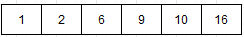Step 2: Consider two pointers, low = 0 and high = N - 1, where N is the size of the array.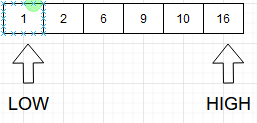Step 3: Find the mid, i.e. mid = (low + high) / 2. Here, mid = (0 + 6) / 2 = 3.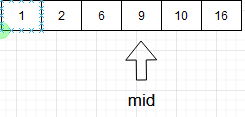Step 4: Now, check for three conditions:

• Compare if A[mid] = 2
• Compare if A[mid] > 2
• Compare if A[mid] < 2
• It can be observed that, A[mid] = 9, which is > 2, therefore, we shift, high = mid - 1.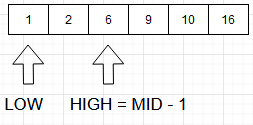Step 5: If A[mid] < 2, shift low = mid + 1.

Step 6: Repeat Steps 3 to Step 5, until the search area is limited to just one element i.e. low = high.

Step 7: The value 2 is found.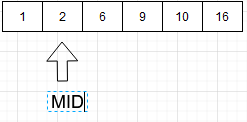### Binary Search Implementations

Binary Search can be implemented in mainly two ways:

• Recursive method
• Iterative method

Let us first discuss the Recursive method.

### Recursive Method

``````
int bSearch(int arr[], int left, int right, int target) {
if (right >= left) {
int mid = left + (right - left) / 2;

if (arr[mid] == target)
return mid;

if (arr[mid] > target)
return binarySearch(arr, left, mid - 1, target);

return binarySearch(arr, mid + 1, right, target);
}
return -1;
}``````
``````
class BinarySearch {
int bSearch(int arr[], int left, int right, int target) {
if (right >= l) {
int mid = l + (r - l) / 2;

if (arr[mid] == target)
return mid;

if (arr[mid] > target)
return binarySearch(arr, left, mid - 1, target);

return binarySearch(arr, mid + 1, r, x);
}
return -1;
}
}
``````
``````
def bSearch(arr, left, right, target):
if right >= left:

mid = left + (right - left) // 2

if arr[mid] == target:
return mid

elif arr[mid] > target:
return binarySearch(arr, left, mid-1, target)

else:
return binarySearch(arr, mid + 1, right, target)

else:
return -1

``````

Time Complexity :

• Best Case Complexity : O(logN)
• Average Case Complexity : O(logN)
• Worst Case Complexity : O(logN)

### Iterative Method

``````
int bSearch(int arr[], int left, int right, int target) {
while (left <= right) {
int m = left + (right - left) / 2;

if (arr[m] == target)
return m;

if (arr[m] < target)
left = m + 1;

else
right = m - 1;
}

return -1;
}

``````
``````
class BinarySearch {
int bSearch(int arr[], int left, int right, int target)
{
int left = 0, right = arr.length - 1;
while (left <= right) {
int m = left + (right - left) / 2;

if (arr[m] == target)
return m;

if (arr[m] < target)
left = m + 1;

else
right = m - 1;
}
return -1;
}
}
``````
``````
def bSearch(arr, left, right, target):
while left <= right:

mid = left + (right - left) // 2

if arr[mid] == target:
return mid

elif arr[mid] < target:
left = mid + 1

else:
right = mid - 1

return -1

``````

Time Complexity :

• Best Case Complexity : O(logN)
• Average Case Complexity : O(logN)
• Worst Case Complexity : O(logN)

Walkthrough Examples :

## Serious about Learning Programming ?

Learn this and a lot more with Scaler Academy's industry vetted curriculum which covers Data Structures & Algorithms in depth.
Examples

## Binary Search Problems

Simple binary search
Problem Score Companies Time Status
Search in Bitonic Array! 100 52:26
Smaller or equal elements 200
32:26
Matrix Search 250 36:51
Search for a Range 250 42:02
Sorted Insert Position 250
25:03
Problem Score Companies Time Status
Matrix Median 225 77:24
Square Root of Integer 275 40:32
Allocate Books 350 68:01
Painter's Partition Problem 350 81:38
Red Zone 400
50:06
Search step simulation
Problem Score Companies Time Status
Implement Power Function 275
59:44
Simple Queries 300
64:28
Sort modification
Problem Score Companies Time Status
Median of Array 325 87:05
Rotated Sorted Array Search 325 56:53

Problem Score Companies Time Status
Capacity To Ship Packages Within B Days 200 33:18Free Mock Assessment
Help us know you better for the best experience
Current Employer *
Enter company name